# Telling Time to the Minute: Before and After the Hour

Content Telling Time to the Minute: Before and After the Hour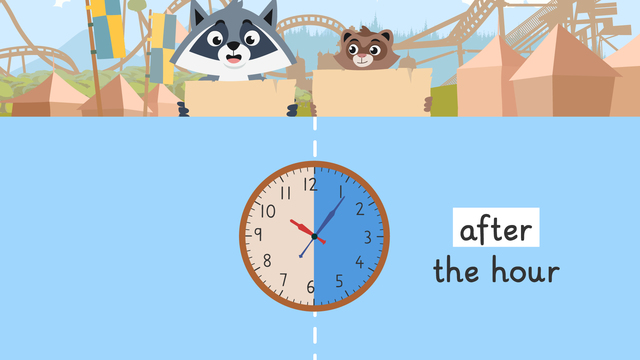Rating

Ø 4.7 / 3 ratings
The authorsTeam Digital
Telling Time to the Minute: Before and After the Hour
CCSS.MATH.CONTENT.4.MD.A.1

## Telling Time to the Minute

Did you know that youcan use the expressions before the hour and after the hour to tell time to the minute? Why is it important to tell time to the nearest minute? Since there are a few different ways to tell time, is it important to know how to tell time to the nearest minute in every way possible so that you’re able to express yourself precisely. How do you know which expression to use when you tell and write time to the nearest minute? In the following, we will look at exactly how telling time to the nearest minute works.

## Telling Time – After the Hour

Since there are 60 minutes in one hour, we can divide the clock in half at the 30 minute mark. After the hour means how many minutes have passed in the hour. We use the expression after the hour to tell time when the minute hand is on the right side of the clock.

Once you identify the minute hand on the right side of the clock, you use skip counting to count the minutes that have passed in the hour. Lastly, you determine the hour by identifying the last number the hour hand has passed.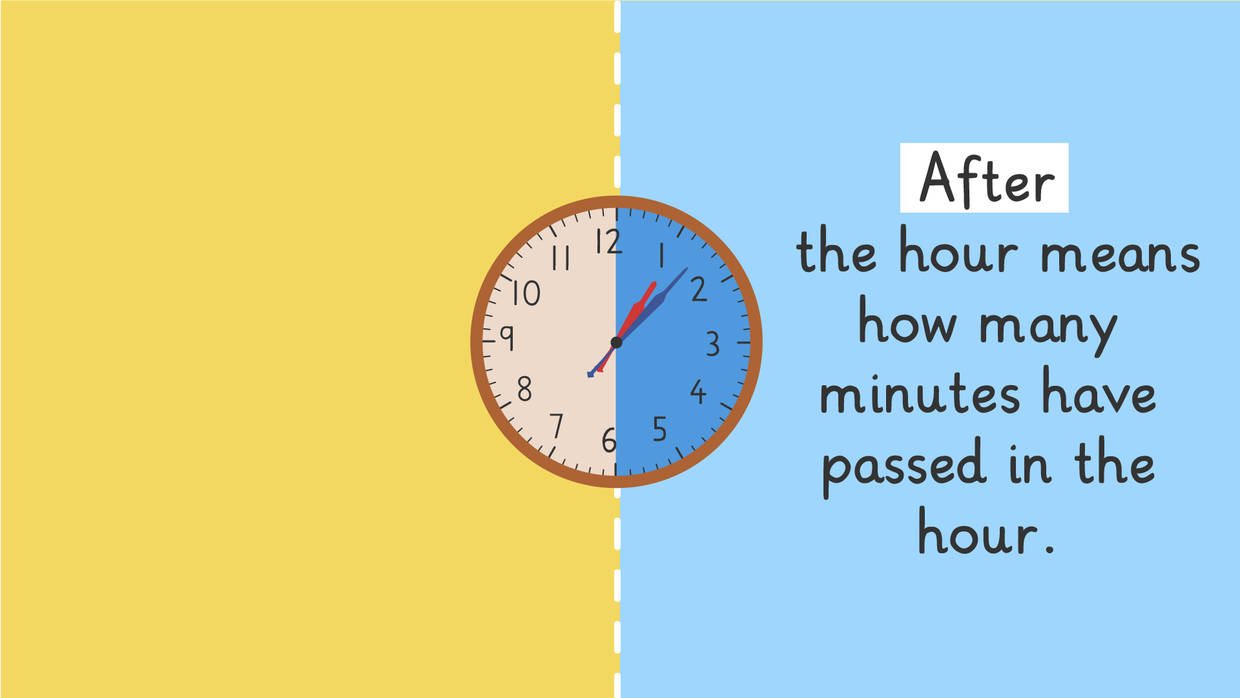## Telling Time – Before the Hour

Before the hour means how many minutes are left before the next hour. We use the expression before the hour to tell time when the minute hand is on the left side of the clock.

Once you identify the minute hand on the left side of the clock, you use skip counting to count how many minutes are left before the next hour. Lastly, you determine the hour by identifying the last number the hour hand has passed.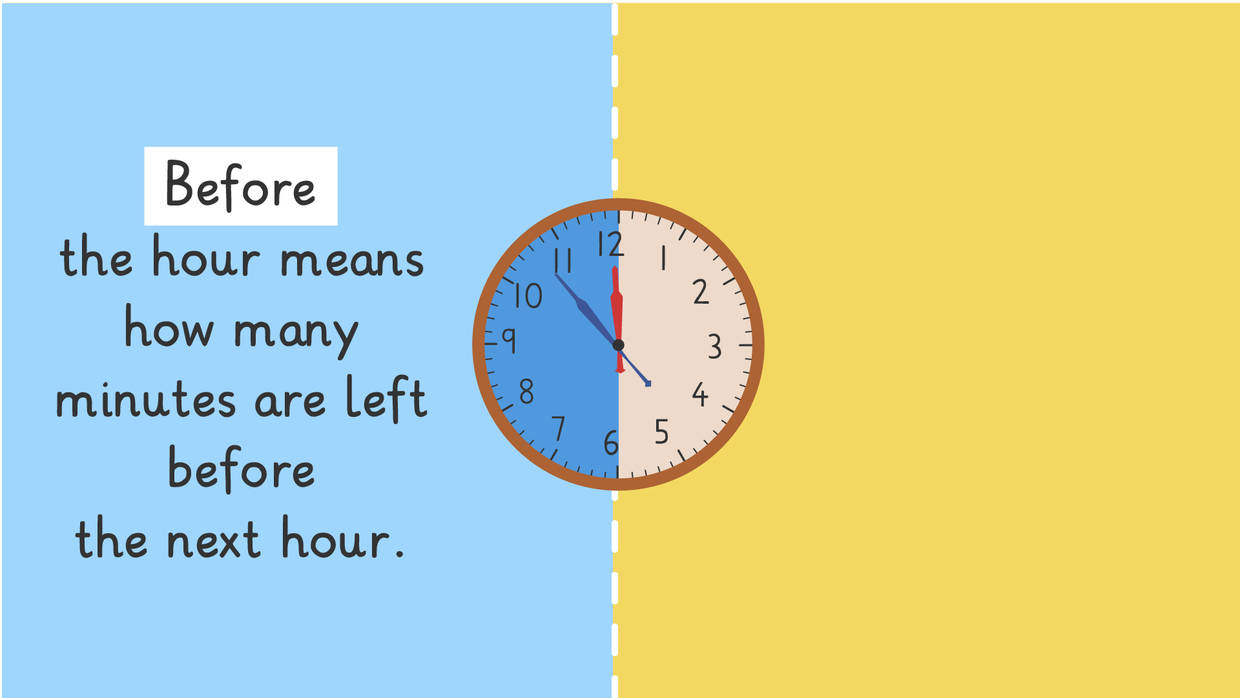## Telling time to the Minute – Example

How can we tell time to the nearest minute using before the hour and after the hour? The clock below shows what time the Monster Truck show starts, but how do you tell time to the minute using before the hour or after the hour?Let’s start by asking, is the minute hand on the right side of the clock or the left side? In this example, the minute hand is on the right side so we will use the expression after the hour.

Next, we will count how many minutes have passed in the hour so far. If you count the tick marks on the clock from the beginning of the hour to where the minute hand is, you will count six, so we know it is six minutes after the hour.

Lastly, we can determine the hour by looking at the hour hand. The hour hand is on ten, so the Monster Truck show starts at six minutes after ten.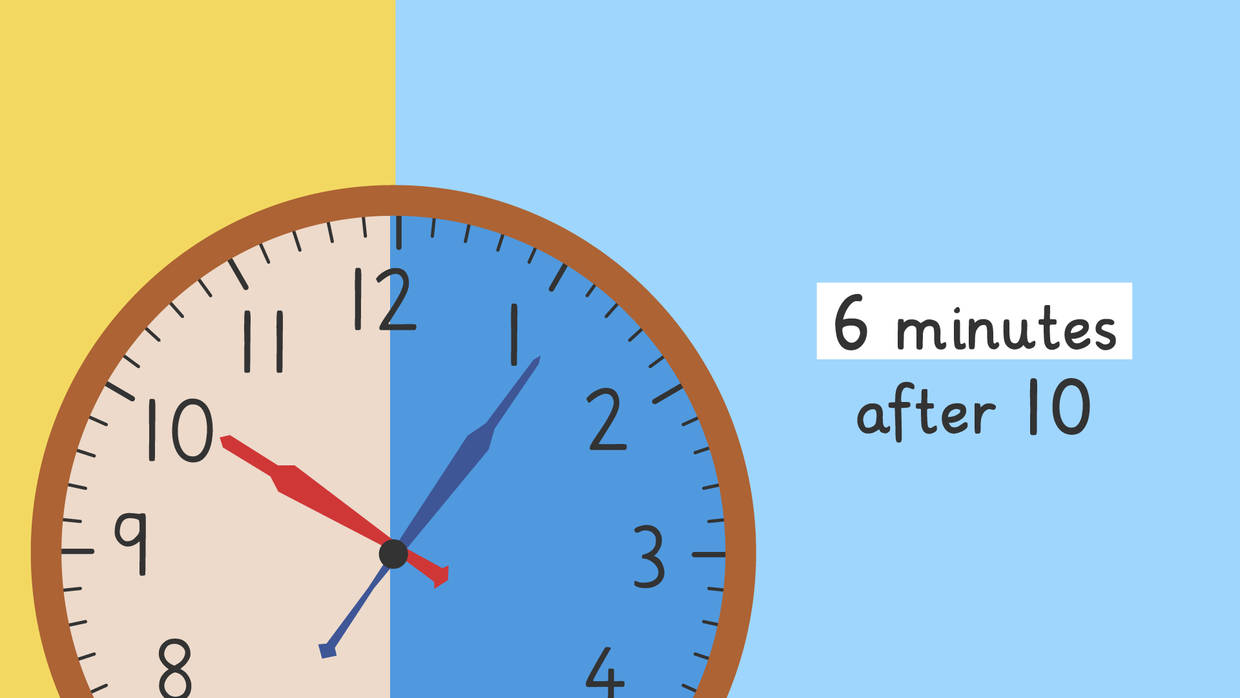Now let’s see what time the Amazing Acrobats show starts. Do we use the expression before the hour or after the hour?Let’s start by asking, is the minute hand on the right side of the clock or the left side? In this example, the minute hand is on the left side so we will use the expression before the hour.

Next, we will count how many minutes are left before the next hour. If you count the tick marks on the clock from where the minute hand is to the end of the hour, you will count seven, so we know it is seven minutes before the next hour.

Lastly, we can determine the hour by looking at the hour hand. The last number the hour hand passed is eleven, so the Amazing Acrobats show starts at seven minutes before twelve.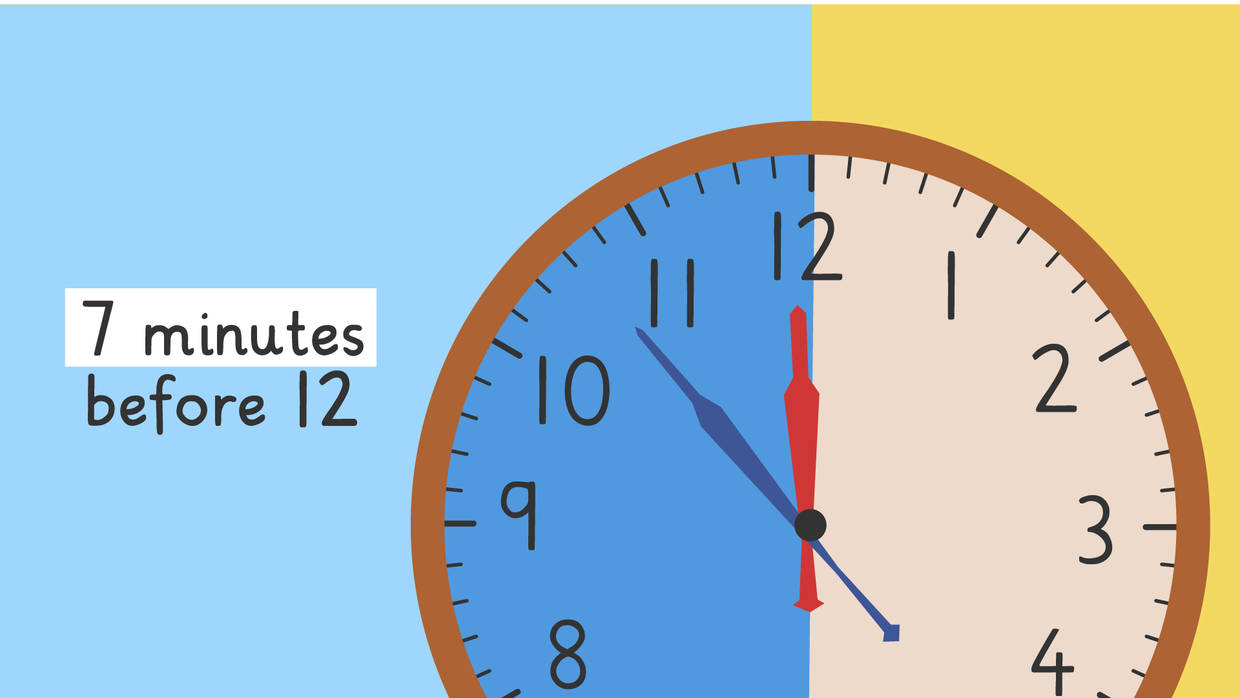## Telling Time to the Minute – Summary

How can we tell time to the minute using before the hour and after the hour? Remember, when we tell time to the minute using the expressions before the hour and after the hour:

Step # What to do
1 Identify which expression to use
2 Count the minutes
3 Determine the hour

Be sure to check out our interactive exercises, worksheets and further activities on telling time to the minute for more practice. You find them after watching the video.

### TranscriptTelling Time to the Minute: Before and After the Hour

Zuri and Freddie got to The Bee's Knees Adventure Park and are looking at a park map to try and make a plan for their day. They know they only have time to make it to one of the live shows if they want to ride all the rides. "Should we try to see the Amazing Acro Bats at seven minutes before twelve?"
"Do you mean 11:53?" "Actually you can also use the expressions 'before the hour' and 'after the hour' to tell time." "Telling Time: Before the Hour & After the Hour" In order to tell time using the expressions "before the hour" and "after the hour", you have to decide which one to use first. Since there are sixty minutes in one hour, we can divide our clock in half at the thirty minute mark. AFTER the hour means how many minutes have passed in the hour. BEFORE the hour means how many minutes are left before the next hour. "When we tell time using the expression AFTER the Hour, that means the blue minute hand is on the RIGHT side of the clock." When we tell time using the expression BEFORE the Hour, that means the blue minute hand is on the LEFT side of the clock. Let's look at the Amazing Acro Bats show time. Which side of the clock is the minute hand on?

The blue minute hand is on the LEFT side so we can use the expression BEFORE the hour. Now, let's count how many minutes we have until the next hour. Remember, each little line marks one minute. One, two, three, four, five, six, seven! There are seven minutes BEFORE the next hour. Now, let's determine the hour. Since the red hour hand is right before the twelve, we know it is seven minutes BEFORE twelve.

Now, let's identify which expression to use for the Monster Truck Show. Which side of the clock is the minute hand on?

The minute hand is on the RIGHT side so we can use the expression AFTER the hour. Now, let's count how many minutes have passed in the hour. One, two, three, four, five, six! It is six minutes AFTER the hour. Now, let's determine the hour. Since the hour hand is on the ten, we know it is six minutes AFTER ten.

Okay, let's see if you can identify which expression to use for the Bee Witchery Magic Show on your own. The first step is to identify which expression to use. You have to see which side of the clock the minute hand is on. Did you choose BEFORE the hour or AFTER the hour?

Here, we use the expression BEFORE the hour, because the minute hand is on the LEFT side of the clock. The second step is, to count how many minutes have passed in the hour OR how many minutes until the next hour. What do you think?

How many minutes are there before the next hour?

Five, ten, fifteen, sixteen, seventeen. The third step is to determine the hour. When does the Bee Witchery Magic show start?

The Bee Witchery Magic show starts seventeen minutes before two. Zuri and Freddie now know each show time, so they can decide which one to watch! Remember, the first step is to identify which expression to use. To do this, ask yourself 'which side of the clock is the minute hand on?' When we tell time using the expression AFTER the Hour, that means the minute hand is on the RIGHT side of the clock. When we tell time using the expression BEFORE the Hour, that means the minute hand is on the LEFT side of the clock. The second step is, to count the minutes. The third step is to determine the hour. Speaking of the show times, I wonder which show Zuri and Freddie chose?

## Telling Time to the Minute: Before and After the Hour exercise

Would you like to apply the knowledge you’ve learned? You can review and practice it with the tasks for the video Telling Time to the Minute: Before and After the Hour.
• ### Sort the steps to tell time before and after the hour.

Hints

Think back! What should you do before you count the minutes before the hour?

Before you determine the hour, what must you do?

Solution
1. Divide clock in half.
2. Which side is the minute hand on?
3. Count the minutes before the hour.
4. Lastly, determine the hour.
• ### Find the clock that matches the time.

Hints

Remember after the hour means that the minute hand is on the right, and before the hour means that the minute hand is on the left.

Count the minutes before or after the hour.

Determine the hour by looking if the hour hand is before the next hour or after the next hour.

Solution

• The minute hand is on the left side, so we can use the expression before the hour.
After skip counting and counting up the minutes until the next hour, we get 12 minutes. Since the hour hand is right before 6, we know it is 12 minutes before 6. _____________________________________________________________________________________ For the other clocks:
• The minute hand is on the right side, so we can use the expression after the hour.
After skip counting and counting up the minutes after the hour, we get 22 minutes. Since the hour hand is right after 3, we know it is 22 minutes after 3.
• The minute hand is on the left side, so we can use the expression before the hour.
After skip counting and counting up the minutes until the next hour, we get 7 minutes. Since the hour hand is right before 12, we know it is 7 minutes before 12.
• The minute hand is on the right side, so we can use the expression after the hour.
After skip counting and counting up the minutes after the hour, we get 6 minutes. Since the hour hand is right after 10, we know it is 6 minutes after 10.

• ### Match the time to the clock.

Hints

Remember, after the hour means that the minute hand is on the right, and before the hour means that the minute hand is on the left.

Count the minutes before or after the hour.

Determine the hour by looking if the hour hand is before the next hour or after the next hour.

Solution

The first clock:

• The minute hand is on the right side, so we can use the expression after the hour.
• After skip counting and counting up the minutes after the hour, we get 18 minutes.
• Since the hour hand is right after 4, we know it is 18 minutes after 4.
_____________________________________________________________________________________ For the other clocks:
The second clock:
• The minute hand is on the left side, so we can use the expression before the hour.
• After skip counting and counting up the minutes until the next hour, we get 28 minutes.
• Since the hour hand is right before 10, we know it is 28 minutes before 10.
The third clock:
• The minute hand is on the right side, so we can use the expression after the hour.
• After skip counting and counting up the minutes after the hour, we get 8 minutes.
• Since the hour hand is right after 6, we know it is 8 minutes after 6.
The last clock:
• The minute hand is on the left side, so we can use the expression before the hour.
• After skip counting and counting up the minutes until the next hour, we get 17 minutes.
• Since the hour hand is right before 8, we know it is 17 minutes before 8.

• ### What time is it before or after the hour?

Hints

Remember, after the hour means that the minute hand is on the right, and before the hour means that the minute hand is on the left.

Count the minutes before or after the hour.

Determine the hour by looking if the hour hand is before the next hour or after the next hour.

Solution

The first clock:

• The minute hand is on the left side, so we can use the expression before the hour.
• After skip counting and counting up the minutes until the next hour, we get 18 minutes.
• Since the hour hand is right before 2, we know it is 18 minutes before 2.
_____________________________________________________________________________________ For the other clocks:
The second clock:
• The minute hand is on the right side, so we can use the expression after the hour.
• After skip counting and counting up the minutes after the hour, we get 16 minutes.
• Since the hour hand is right after 10, we know it is 16 minutes after 10.
The third clock:
• The minute hand is on the left side, so we can use the expression before the hour.
• After skip counting and counting up the minutes until the next hour, we get 3 minutes.
• Since the hour hand is right before 6, we know it is 3 minutes before 6.
The last clock:
• The minute hand is on the right side, so we can use the expression after the hour.
• After skip counting and counting up the minutes after the hour, we get 8 minutes.
• Since the hour hand is right after 8, we know it is 8 minutes after 8.

• ### Identify which expression to use.

Hints

Remember, the clock is divided in half at the 30 minute mark.

Remember, after the hour means that the minute hand is on the right, and before the hour means that the minute hand is on the left.

Solution

The clock is divided in half at the 30 minute mark. Before the hour is used when the minute hand is on the left. After the hour is used when the minute hand is on the right.

• The first clock is after the hour because the minute hand is on the right.
• The second clock is before the hour because the minute hand is on the left.
• The third clock is after the hour because the minute hand is on the right.
• The last clock is before the hour because the minute hand is on the left.

• ### Which times are wrong?

Hints

Remember, after the hour means that the minute hand is on the right, and before the hour means that the minute hand is on the left.

Count the minutes before or after the hour.

Determine the hour by looking if the hour hand is before the next hour or after the next hour.

Solution

The second time is 7 minutes before 6, not 7 minutes before 5.

• The minute hand is on the left side, so we can use the expression before the hour.
• After skip counting and counting up the minutes until the next hour, we get 7 minutes.
• Since the hour hand is right before 6, we know it is 7 minutes before 6.
________________________________________________________________________________________________________ For the other clocks:
The first time is 26 minutes after 1, not 26 minutes before 1.
• The minute hand is on the right side, so we can use the expression after the hour.
• After skip counting and counting up the minutes after the hour, we get 26 minutes.
• Since the hour hand is right after 1, we know it is 26 minutes after 1.
The third time is 16 minutes after 8, not 16 minutes before 4.
• The minute hand is on the right side, so we can use the expression after the hour.
• After skip counting and counting up the minutes after the hour, we get 16 minutes.
• Since the hour hand is right after 8, we know it is 16 minutes after 8.
The last time is 3 minutes after 11, not 3 minutes after 12.
• The minute hand is on the right side, so we can use the expression after the hour.
• After skip counting and counting up the minutes after the hour, we get 3 minutes.
• Since the hour hand is right after 11, we know it is 3 minutes after 11.